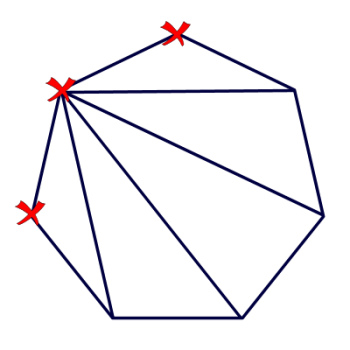Games
Problems
Go Pro!Ninth grader Michaela wants to know how you can "find the number of diagonals [in a polygon] drawn from a single vertex."

In answering this question, I'd like to point out that my diagram shows a convex polygon. If the polygon is concave, some of the diagonals will lie outside the polygon, but we would still call them diagonals.

If you look at the diagram to the right, you'll see that I've marked three vertices with an X.  Why? Because those are the vertices we can't draw a diagonal to.  You can't draw a diagonal from a vertex to itself, and you can't draw a diagonal from a vertex to its neighboring vertex. After all, drawing a line between two neighboring vertices isn't drawing a diagonal; it's drawing a side!

So, there are seven vertices in my polygon, and we can't draw diagonals to three of them, so that means we can draw diagonals to 7 - 3 = 4 vertices.

Can we generalize that? Of course! No matter how many sides the polygon has, there will always be 3 vertices you can't draw a diagonal to.

So if it has n vertices? You can draw n - 3 diagonals from any vertex.

As an added bonus (this goes beyond what you asked, Michaela!), this is how we get the formula for the number of diagonals in a polygon.

There are n vertices, and we can draw n - 3 diagonals from each one, making a total of n(n - 3) diagonals. Except...wait a minute! We've counted each diagonal TWICE (because we counted both endpoints!). So we need to divide that by two:

Total diagonals = n(n - 3)/2.

Professor PuzzlerLike us on Facebook to get updates about new resources